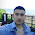Bloom Filters - Explained

The Bloom filter is a space efficient, probabilistic data structure, designed to test the membership of elements to a set. The trade-off for being a space efficient data structure is it may return false positives, but always returns definite negatives: Meaning Bloom filters can accurately test an element for non-membership to a set, but can only with probability test an element for membership. Bloom filters find application in circumstances where testing for non-membership saves resources such as calls to a web server, checking a proxy cache. Google uses Bloom filters in the Chrome browser as a preliminary check for malicious URL's.

Internally Bloom filters use a binary-array, and multiple hash functions. Lets say for instance we have a bit-array of a 100 elements, and 3 hash functions. We want to insert the word "Singapore" into the filter, so we pass it through hash 1 - returns 33; hash 2 - returns 7; hash 3 - returns 22. Next we go to each of those indices of the array and set them to 1, we are done and the word has been included for testing. To test a word for membership, we pass the word through all three hash functions, get the three hashes and check the corresponding indices of the bit-array. If all 3 indices are set to 1, then its a probable true; if any one of those indices are set to zero, it's a definite false. Below is an example...

The efficiency of Bloom filter relies upon the number of bits in the array, the number of hash functions, and most importantly the quality of the hash functions. In a real world example, we would use 14 hash functions, and a binary-array of millions of elements. xxHash is one of the higher quality hash functions available, other examples are MurmurHash, FNV Hash, Jenkins Hashes, pseudo-number generators will also do. Uniform Distribution of hash functions being a key factor in quality. Here are the formulas invloved in the designing of a bloom filter:

m - number of elements in bit array
k - number of hash functions
n - number of items in collection
p - desired false positive probability // 0.0 - 1.0
^ - power

m = -((n*ln(p))/(ln(2)^2));
k = (m/n) * ln(2);
n = (m * ln(2))/k;
p = e^(-(m/n) * (ln(2)^2)); // estimated probability of false positives
// lets do a test, i know n = 1000,
// and i desire a p = 0.01 :
m = 9585.058 = 9586
k = 6.644 = 7

// so for 100 items in a collection,
// a desired probability of 1% false positives
// you need a bit array of 9586 elements and 7 hash functions */

Thank you for reading - I'll keep on editing this tutorial in the future.

http://en.wikipedia.org/wiki/Bloom_filter
http://www.jasondavies.com/bloomfilter/

1.Thank you for this. What a simple and profound idea!

2.Magnificient Meerkat13 January 2015 at 13:22

My gratitude as well, I just learnt something.

3.Great blog! Thank you!

4.Awesome! The way you explain concepts is just great

5.Awesome! The way you explain concepts is just great

6.I'am glad to read the whole content of this blog and am very excited.Thank you.

หีฟิต